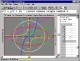Graphmatica 2.4 powerful, easy-to-use, equation plotter with numerical and calculus features SharewareCalculus 1.1.2 Calculus brings you an useful calculator that besides the usual converting functions (Temperature, Pressure, Speed etc. Shareware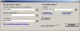PEEI 4.0.0.1 Solver of systems of partial differential equations. FreewareZ-Plot 1.02 2D / 3D function plotter that is able to calculate with complex numbers Freeware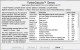FC Compiler 6 FC-Compiler (tm) is a Calculus (level) Compiler, helps Tweak parameters. FreewareExtractor 1.0 Extractor is an application of scientific calculus of high precision. SharewareAbacus Math Writer 4.0 For publishing Mathematical and Scientific equations. Shareware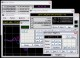Kalkulator 2.41 Advanced Sci/Eng calculator, from simple expressions to differential equations SharewareODEcalc 6.1 ODEcalc (tm): Ordinary Differential Equation (ODE) Calculator! Solves ODEs Freeware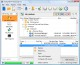Backup4all Professional 8.5.264 Customizable backup program. Full, incremental, differential and mirror backups Shareware